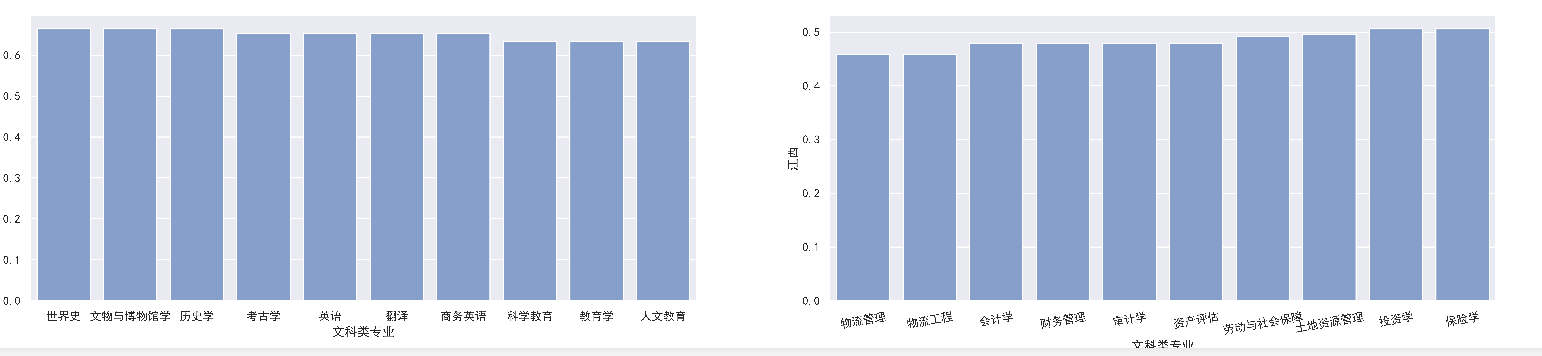python 用matplotlib绘图，如何让多个子图的横轴标签全部旋转

fig,axes=plt.subplots(1,2,figsize=(25, 5))

``````ax1 = sns.barplot(x="文科类专业", y=i, data=df1后,
color="cornflowerblue",saturation=0.5,ci=0,ax=axes)  ##画一个图

ax2 = sns.barplot(x="文科类专业", y=i, data=df1前,
color="cornflowerblue",saturation=0.5,ci=0,ax=axes)  ##画一个图
plt.xticks(rotation=10)    ##x轴名字倾斜
``````1个回答